# 13.2 Arithmetic sequences  (Page 6/8)

 Page 6 / 8

For the following exercises, use the explicit formula to write the first five terms of the arithmetic sequence.

${a}_{n}=24-4n$

First five terms: $20,16,12,8,4.$

${a}_{n}=\frac{1}{2}n-\frac{1}{2}$

For the following exercises, write an explicit formula for each arithmetic sequence.

${a}_{n}=\left\{3,5,7,...\right\}$

${a}_{n}=1+2n$

${a}_{n}=\left\{32,24,16,...\right\}$

${a}_{n}=-105+100n$

${a}_{n}=1.8n$

${a}_{n}=\left\{-18.1,-16.2,-14.3,...\right\}$

${a}_{n}=\left\{15.8,18.5,21.2,...\right\}$

${a}_{n}=13.1+2.7n$

${a}_{n}=\left\{0,\frac{1}{3},\frac{2}{3},...\right\}$

${a}_{n}=\frac{1}{3}n-\frac{1}{3}$

${a}_{n}=\left\{-5,-\frac{10}{3},-\frac{5}{3},\dots \right\}$

For the following exercises, find the number of terms in the given finite arithmetic sequence.

There are 10 terms in the sequence.

${a}_{n}=\left\{1.2,1.4,1.6,...,3.8\right\}$

${a}_{n}=\left\{\frac{1}{2},2,\frac{7}{2},...,8\right\}$

There are 6 terms in the sequence.

## Graphical

For the following exercises, determine whether the graph shown represents an arithmetic sequence.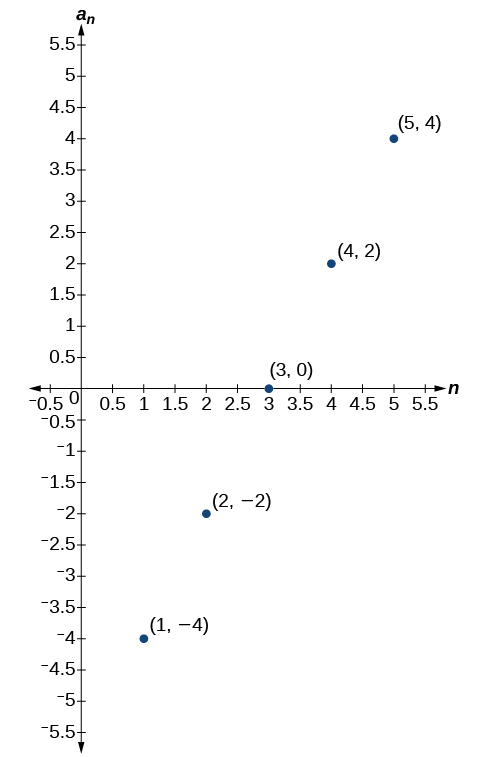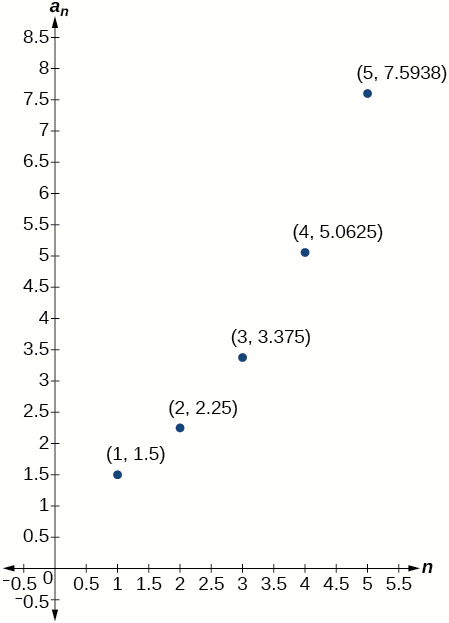The graph does not represent an arithmetic sequence.

For the following exercises, use the information provided to graph the first 5 terms of the arithmetic sequence.

${a}_{1}=0,d=4$

${a}_{1}=9;{a}_{n}={a}_{n-1}-10$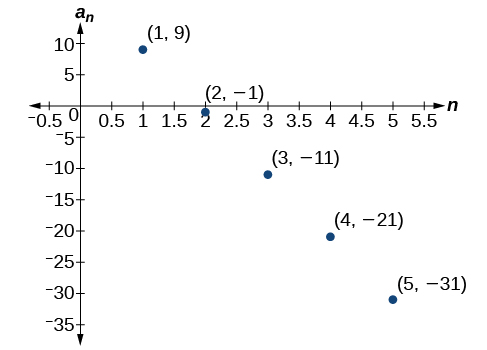${a}_{n}=-12+5n$

## Technology

For the following exercises, follow the steps to work with the arithmetic sequence ${a}_{n}=3n-2$ using a graphing calculator:

• Press [MODE]
• Select SEQ in the fourth line
• Select DOT in the fifth line
• Press [ENTER]
• Press [Y=]
• $n\text{Min}\text{\hspace{0.17em}}$ is the first counting number for the sequence. Set $\text{\hspace{0.17em}}n\text{Min}=1$
• $u\left(n\right)\text{\hspace{0.17em}}$ is the pattern for the sequence. Set $\text{\hspace{0.17em}}u\left(n\right)=3n-2$
• $u\left(n\text{Min)}\text{\hspace{0.17em}}$ is the first number in the sequence. Set $\text{\hspace{0.17em}}u\left(n\text{Min)}=1$
• Press [2ND] then [WINDOW] to go to TBLSET
• Set $\text{\hspace{0.17em}}\text{TblStart}=1$
• Set $\text{\hspace{0.17em}}\Delta \text{Tbl}=1$
• Set Indpnt: Auto and Depend: Auto
• Press [2ND] then [GRAPH] to go to the TABLE

What are the first seven terms shown in the column with the heading $u\left(n\right)\text{?}$

$1,4,7,10,13,16,19$

Use the scroll-down arrow to scroll to $n=50.$ What value is given for $u\left(n\right)\text{?}$

Press [WINDOW] . Set $\text{\hspace{0.17em}}n\text{Min}=1,n\text{Max}=5,x\text{Min}=0,x\text{Max}=6,y\text{Min}=-1,\text{\hspace{0.17em}}$ and $\text{\hspace{0.17em}}y\text{Max}=14.\text{\hspace{0.17em}}$ Then press [GRAPH] . Graph the sequence as it appears on the graphing calculator.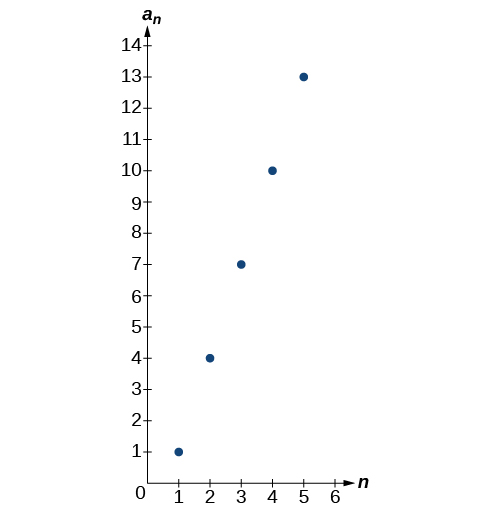For the following exercises, follow the steps given above to work with the arithmetic sequence ${a}_{n}=\frac{1}{2}n+5$ using a graphing calculator.

What are the first seven terms shown in the column with the heading $\text{\hspace{0.17em}}u\left(n\right)\text{\hspace{0.17em}}$ in the TABLE feature?

Graph the sequence as it appears on the graphing calculator. Be sure to adjust the WINDOW settings as needed.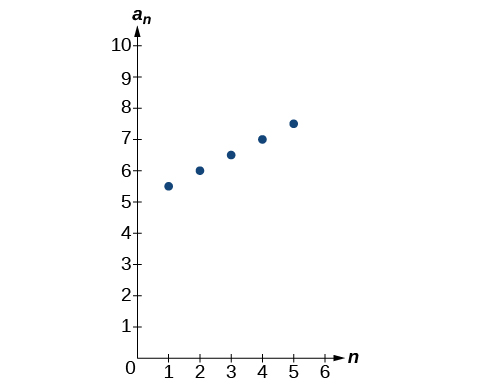## Extensions

Give two examples of arithmetic sequences whose 4 th terms are $9.$

Give two examples of arithmetic sequences whose 10 th terms are $206.$

Answers will vary. Examples: ${a}_{n}=20.6n$ and ${a}_{n}=2+20.4\mathrm{n.}$

Find the 5 th term of the arithmetic sequence $\left\{9b,5b,b,\dots \right\}.$

Find the 11 th term of the arithmetic sequence $\left\{3a-2b,a+2b,-a+6b\dots \right\}.$

${a}_{11}=-17a+38b$

At which term does the sequence $\left\{5.4,14.5,23.6,...\right\}$ exceed 151?

At which term does the sequence $\left\{\frac{17}{3},\frac{31}{6},\frac{14}{3},...\right\}$ begin to have negative values?

The sequence begins to have negative values at the 13 th term, ${a}_{13}=-\frac{1}{3}$

For which terms does the finite arithmetic sequence $\left\{\frac{5}{2},\frac{19}{8},\frac{9}{4},...,\frac{1}{8}\right\}$ have integer values?

Write an arithmetic sequence using a recursive formula. Show the first 4 terms, and then find the 31 st term.

Answers will vary. Check to see that the sequence is arithmetic. Example: Recursive formula: ${a}_{1}=3,{a}_{n}={a}_{n-1}-3.$ First 4 terms: $\begin{array}{ll}3,0,-3,-6\hfill & {a}_{31}=-87\hfill \end{array}$

Write an arithmetic sequence using an explicit formula. Show the first 4 terms, and then find the 28 th term.

what are odd numbers
numbers that leave a remainder when divided by 2
Thorben
1,3,5,7,... 99,...867
Thorben
7%2=1, 679%2=1, 866245%2=1
Thorben
the third and the seventh terms of a G.P are 81 and 16, find the first and fifth terms.
if a=3, b =4 and c=5 find the six trigonometric value sin
pls how do I factorize x⁴+x³-7x²-x+6=0
in a function the input value is called
how do I test for values on the number line
if a=4 b=4 then a+b=
a+b+2ab
Kin
commulative principle
a+b= 4+4=8
Mimi
If a=4 and b=4 then we add the value of a and b i.e a+b=4+4=8.
Tariq
what are examples of natural number
an equation for the line that goes through the point (-1,12) and has a slope of 2,3
3y=-9x+25
Ishaq
show that the set of natural numberdoes not from agroup with addition or multiplication butit forms aseni group with respect toaaddition as well as multiplication
x^20+x^15+x^10+x^5/x^2+1
evaluate each algebraic expression. 2x+×_2 if ×=5
if the ratio of the root of ax+bx+c =0, show that (m+1)^2 ac =b^2m
By the definition, is such that 0!=1.why?
(1+cosA+IsinA)(1+cosB+isinB)/(cos@+isin@)(cos$+isin$)
hatdog
Mark
jaks
Ryan

#### Get Jobilize Job Search Mobile App in your pocket Now!ByBy Madison ChristianBy Anh DaoBy OpenStaxBy Marion CabalfinBy OpenStaxBy David CoreyBy Samuel MaddenBy OpenStaxBy Michael PittBy Courntey Hub